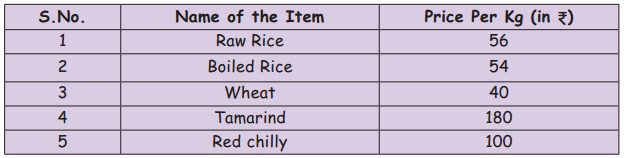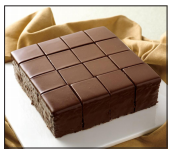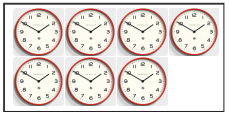Tamilnadu State Board New Syllabus Samacheer Kalvi 4th Maths Guide Pdf Term 3 Chapter 2 Numbers Ex 2.3 Textbook Questions and Answers, Notes.

## Tamilnadu Samacheer Kalvi 4th Maths Solutions Term 3 Chapter 2 Numbers Ex 2.3What else could you frame some more questions based on this table?
i) What is the price of 6 kg of boiled rice?
ii) What is the price of loo g of red chilly?
iii) What is the price of 250 g of tamarind?
iv) What is the price of 2 kg of wheat?
v) What is the sum of the price of 6 kg boiled rice and 1 kg raw rice?Question 1.
Frame word problems, using the picture givenCost of 1 piece of cake ₹ 25